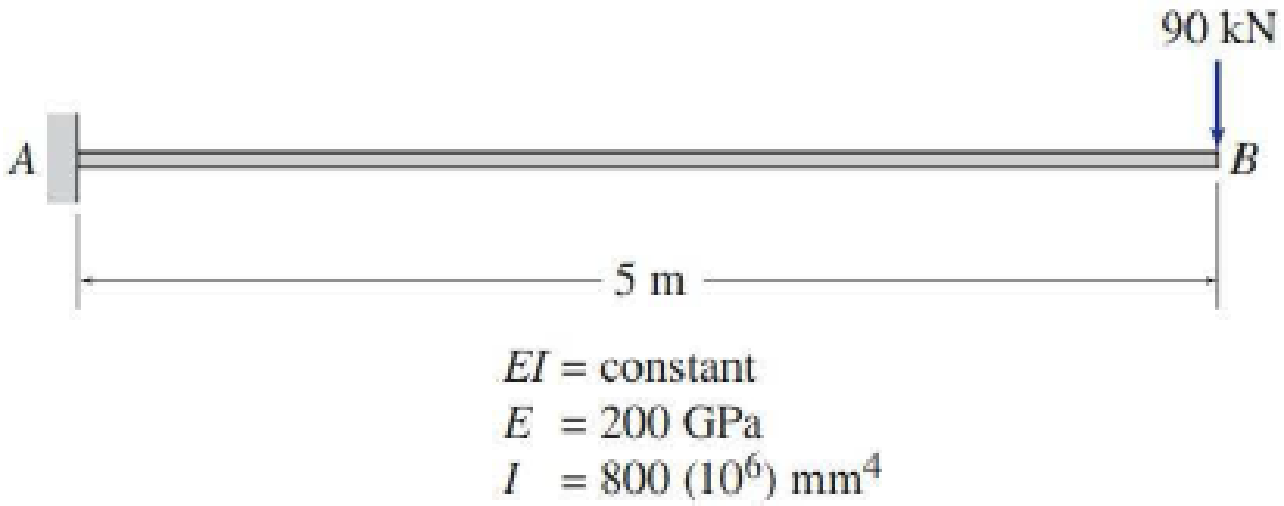# Determine the slope and deflection at point B of the beam shown by the moment-area method. FIG. P6.9, P6.35

#### Solutions

Chapter
Section
Chapter 6, Problem 9P
Textbook Problem
373 views

## Determine the slope and deflection at point B of the beam shown by the moment-area method.FIG. P6.9, P6.35

To determine

Find the slope θB and deflection ΔB at point B of the given beam using the moment-area method.

### Explanation of Solution

Given information:

The Young’s modulus (E) is 200 GPa.

The moment of inertia (I) is 800×106mm4

Calculation:

Consider the flexural rigidity EI of the beam is constant.

Show the given beam as in Figure (1).

Consider clockwise as negative and counterclockwise as positive.

Determine the bending moment at point A using the relation;

MA=P×l

Here, P is the load and l is the distance.

Substitute 90 kN for P and 5 m for l.

MA=90×5=450kNm

Show the M/EI diagram for the given beam as in Figure (2).

Elastic curve:

The sign of M/EI diagram is negative, therefore, the beam bends downward. The support A of the given beam is fixed and the slope at A is zero. Therefore, the tangent to the elastic curve at A is horizontal.

Show the elastic curve diagram as in Figure (3).

The slope at point B can be calculated by evaluating the change in slope between A and B.

Express the change in slope using the first moment-area theorem as follows:

θB=θBA=AreaoftheM/EIbetweenAandB=12×b×h

Here, b is the width and h is the height of the triangle.

Substitute 5.0 m for b and 450EI for h.

θB=θBA=12×5×(450EI)=1,125kNm2EI

Determine the slope at point B using the relation;

θB=1,125kNm2EI

Substitute 200 GPa for E and 800×106mm4 for I.

θB=1,125kNm2(200GPa×(106kN/m21GPa)×800×106mm4×(1m1,000mm)4)=0

### Still sussing out bartleby?

Check out a sample textbook solution.

See a sample solution

#### The Solution to Your Study Problems

Bartleby provides explanations to thousands of textbook problems written by our experts, many with advanced degrees!

Get Started

Find more solutions based on key concepts
What is buoyancy?

Engineering Fundamentals: An Introduction to Engineering (MindTap Course List)

5. Describe a data dictionary and list the types of information it contains.

Systems Analysis and Design (Shelly Cashman Series) (MindTap Course List)

Describe ways a virus infects programs or apps.

Enhanced Discovering Computers 2017 (Shelly Cashman Series) (MindTap Course List)

What are the most popular encryption systems used over the Web?

Principles of Information Security (MindTap Course List)

Describe the basic cash management principles.

Cornerstones of Financial Accounting

If your motherboard supports ECC DDR3 memory, can you substitute non-ECC DDR3 memory?

A+ Guide to Hardware (Standalone Book) (MindTap Course List)

Why must a welding machine's duty cycle never be exceeded?

Welding: Principles and Applications (MindTap Course List)